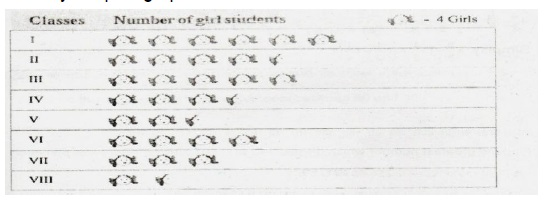# (Download) AISSEE Exam Paper - I Mathematics & Language Ability "Class - VI" - 2016

Disclaimer: This website is NOT associated with CBSE, for official website of CBSE visit - www.cbse.gov.in## "Class - VI" - 2016

ALL INDIA SAINIK SCHOOL ENTRANCE EXAMINATION : 2016
PAPER I – MATHEMATICS AND LANGUAGE ABILITY
CLASS –VI

Time: 2 hrs

Max Marks: 200
Note:-
(1) This question paper contains two parts and you have to answer all questions in both parts.
(2) Part “A” Mathematics has three sections containing 30 questions. Q. Nos 1 to 10carry 2 marks each, Q. Nos 11 to 20 carry 3 marks each and Q. Nos 21 to 30 carry 5 marks each.
(3) Part “B” Language Ability Test contains 10 questions. Q.1 and 2 carry 15 marks each and Q. Nos 5 to 8 carry 5 marks each. The remaining questions carry 10 marks each. In this part you can write your answers in any one of the chosen language only.
(4) Write your answer in Blue/Black/ ink only. Do not use pencil.
(5) All Rough Works is to be done at space provided.

PART-A MATHEMATICS
SECTION – I
(Each question carries two marks)

Q1. Form the smallest and greatest 6-digit numerals by repeating any 2 digits from 7,9,5,4.
Q2. Find the square root of 7921.
Q3. If a man can do a work in 32 days, in how many days will 24 men complete the same work?
Q4. Find the simple interest, ifP = 400, R = 3.65% per annum and time = 150 days.
Q5. Round 48,540 and 23,467 to the nearest 1000 and find the difference.
Q6. Express 804.291 kg as decagrams.
Q7. Find the smallest number which when divided by 12 and 20 leaves no remainder.
Q8. Sonali and Priya are classmates. Sonali completed her homework in 5/6 of an hour and Priya in ¾ of an hour. Who was faster?
Q9. Simplify 6
Q10. Two angles of a quadrilateral are each 900 and remaining two angles are such that one is 3 times the other. Find these two angles.

SECTION –II
(Each question carries three marks)

Q11. Find the ratio of 90 cm to 1.5 m.
Q12. Arrange the following in descending order:

Q13. The number of girl students in each class of a co-educational middle school is depicted by the pictograph:Observe this pictograph and answer the following questions:
(a) Which class has the maximum number of girl students?
(b) Is the number of girls in Class V less than the number of girls in ClassIII?
(c) How many girls are there in Class VII?